Questions in category: 初等几何 (Elementary Geometry)

<   >

## 11. 三角形中构造两条线段, 证明其相等.

Posted by haifeng on 2021-01-26 16:01:39 last update 2021-01-26 16:01:39 | Answers (0) | 收藏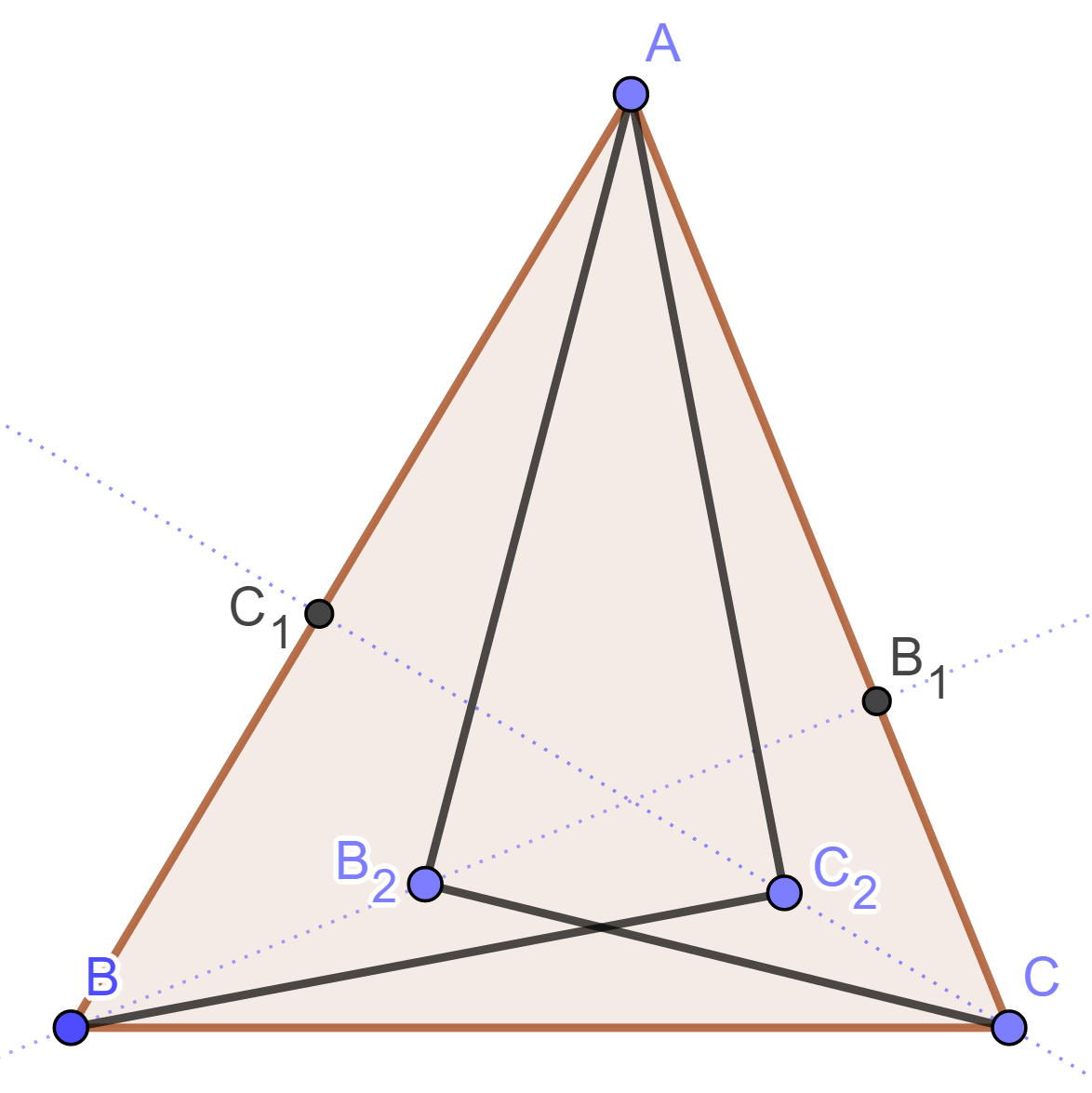## 12. 求下列阴影部分的面积.

Posted by haifeng on 2021-01-26 15:55:19 last update 2021-01-26 15:56:27 | Answers (0) | 收藏

$ABCD$ 和 $EFBG$ 是两个正方形. $ABCD$ 的边长是 10. 求 $\triangle ACE$ 的面积.[Hint] 这个题目并未给出正方形 $EFBG$ 的边长, 事实上 $\triangle ACE$ 的面积与之没有关系.

## 13. 与正方形有关的恒等式

Posted by haifeng on 2021-01-26 10:17:56 last update 2021-01-26 10:57:40 | Answers (0) | 收藏

$ABCD$ 是正方形, 过点 $A$ 的直线分别交直线 $CD$ 与 $BC$ 于 $E$ 和 $F$.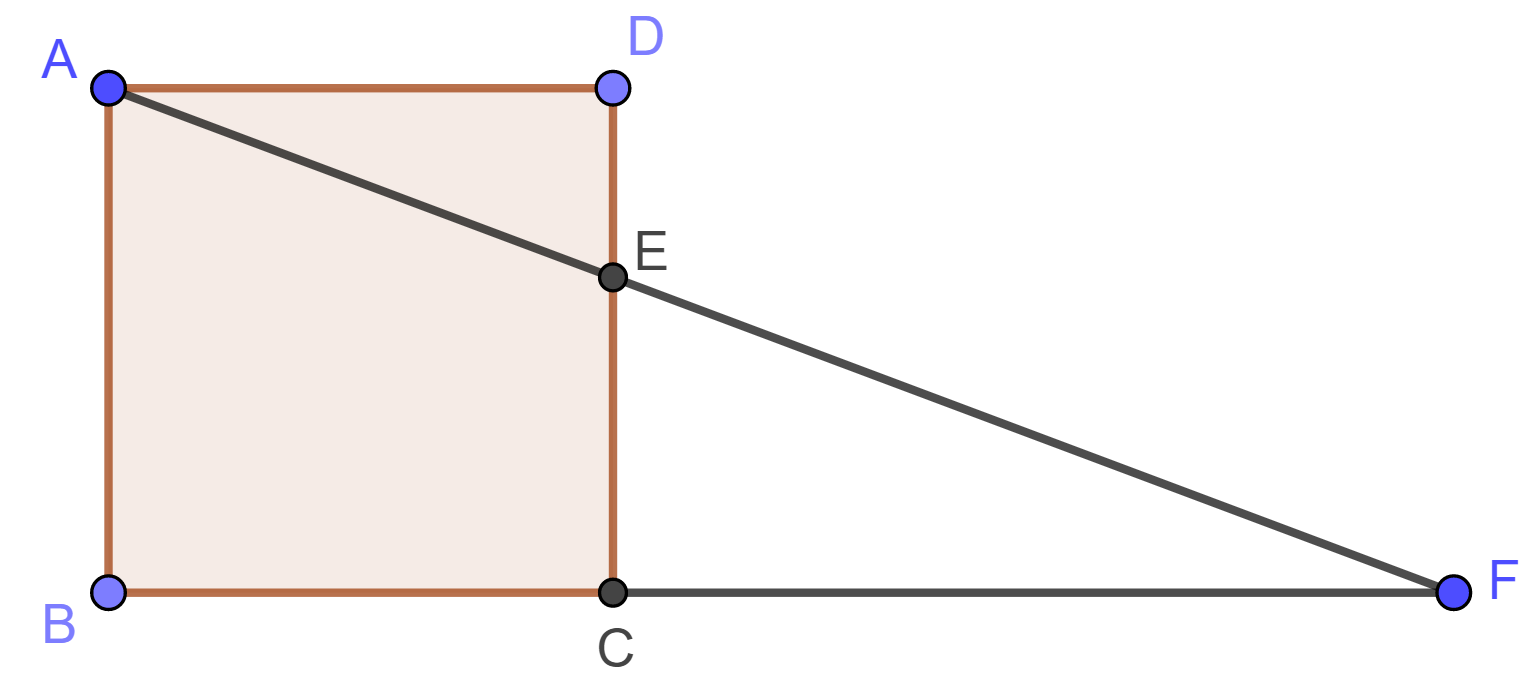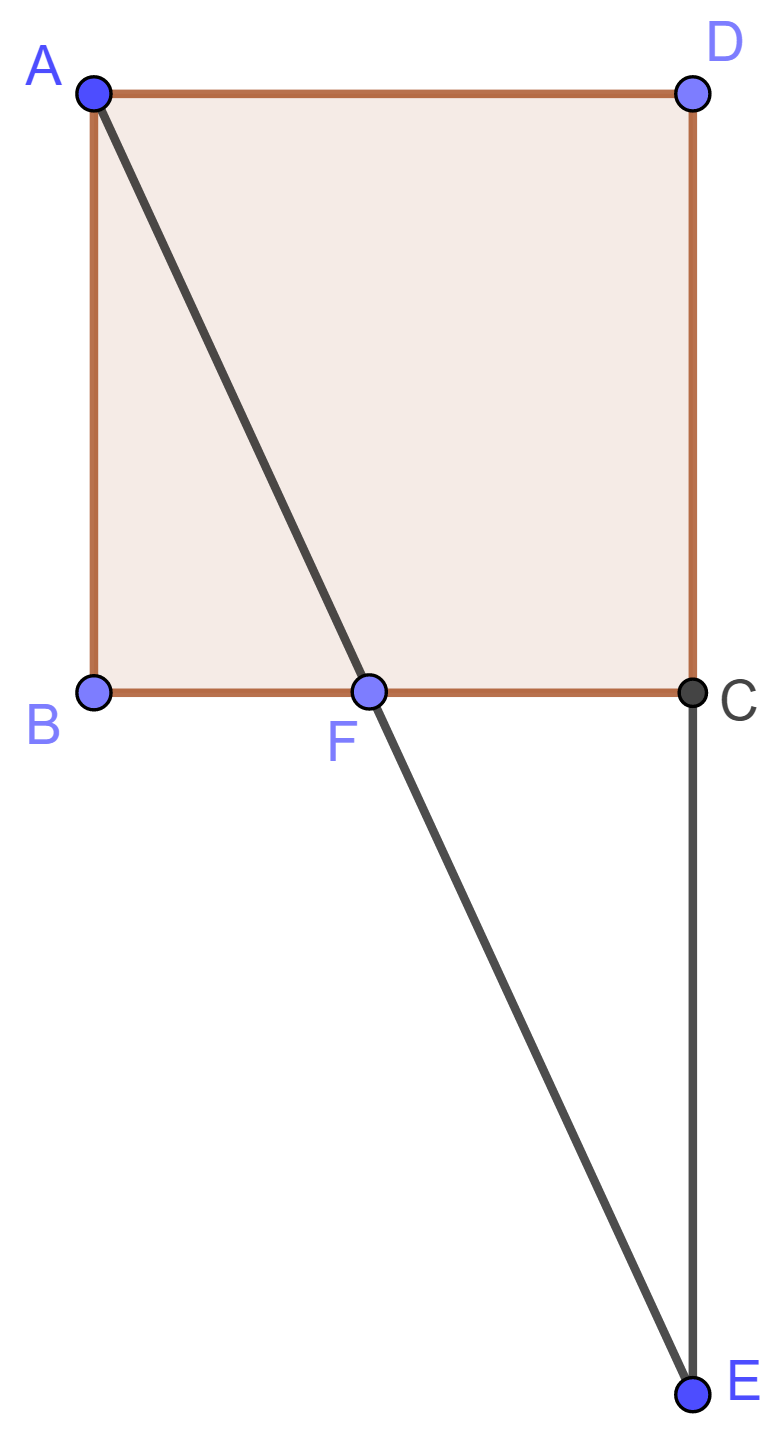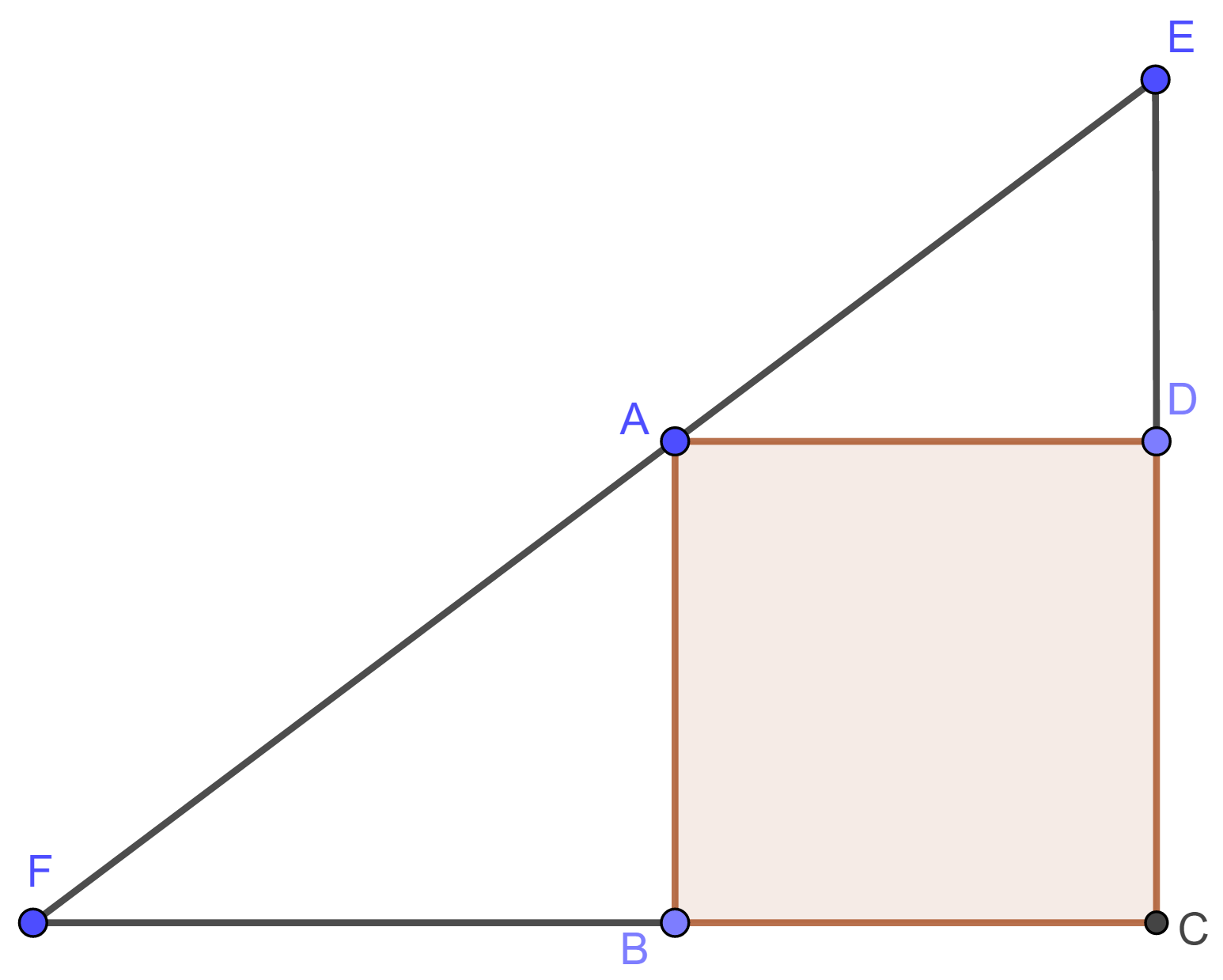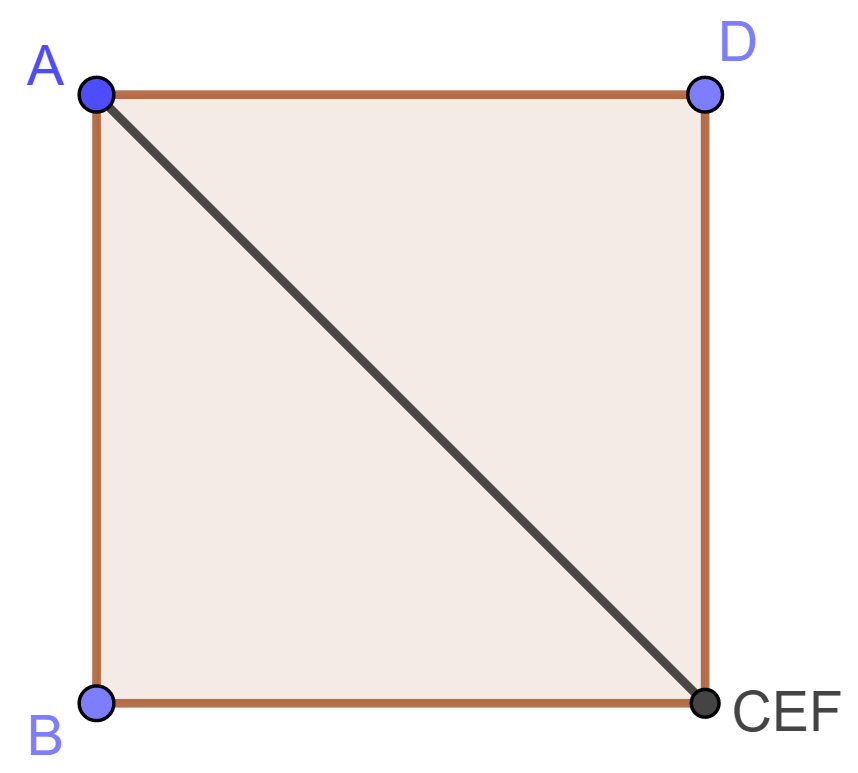## 14. 平行四边形的内接平行四边形一定共心.

Posted by haifeng on 2021-01-25 19:13:28 last update 2021-01-25 20:00:16 | Answers (1) | 收藏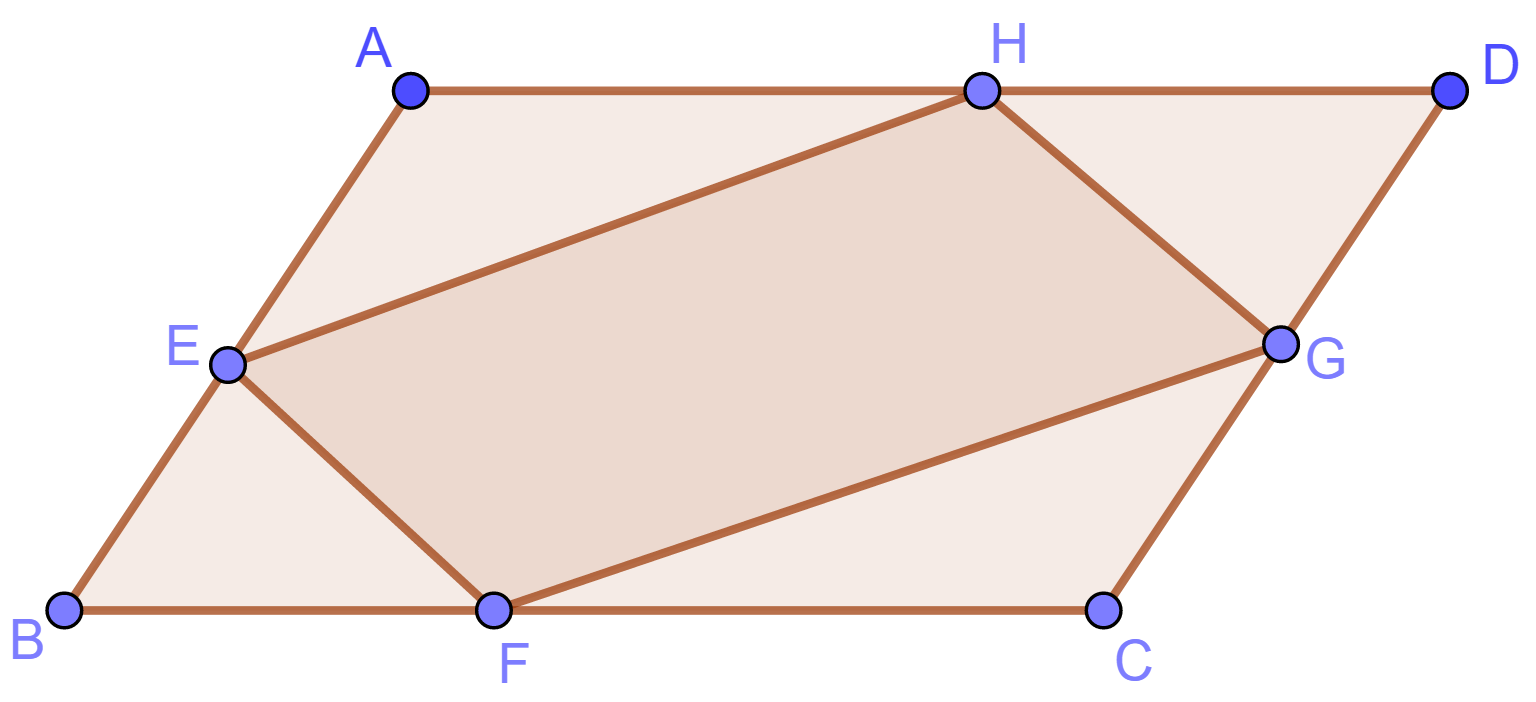【扩展问题1】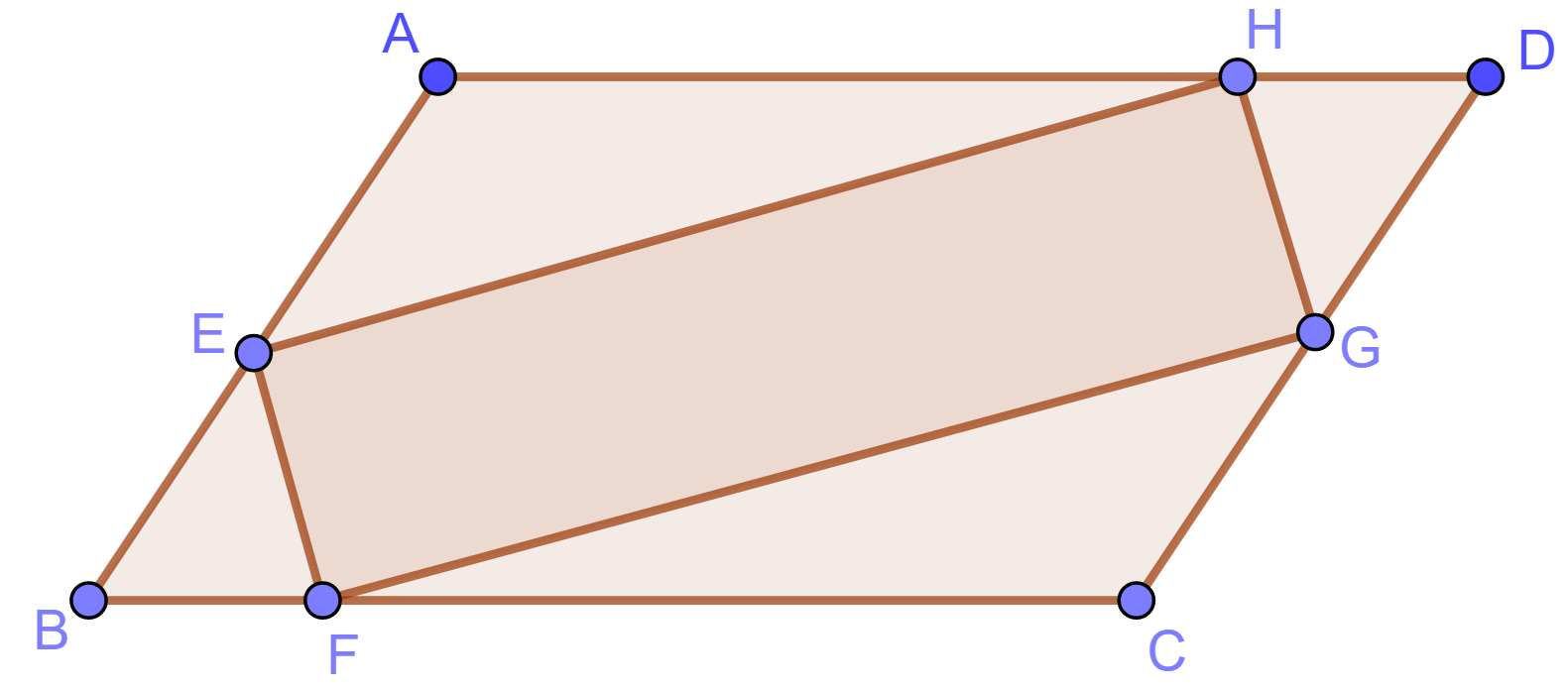(1) 有多少个?
(2) 面积是否都相等?

比如正方形中可以内接无数多个矩形,  存在一个面积最大的内接矩形(实际是正方形).

【扩展问题2】

(1) 对于怎样的 $E$, 最终可以回到出发点?
(2) 如果回不到出发点, 有没有极限点?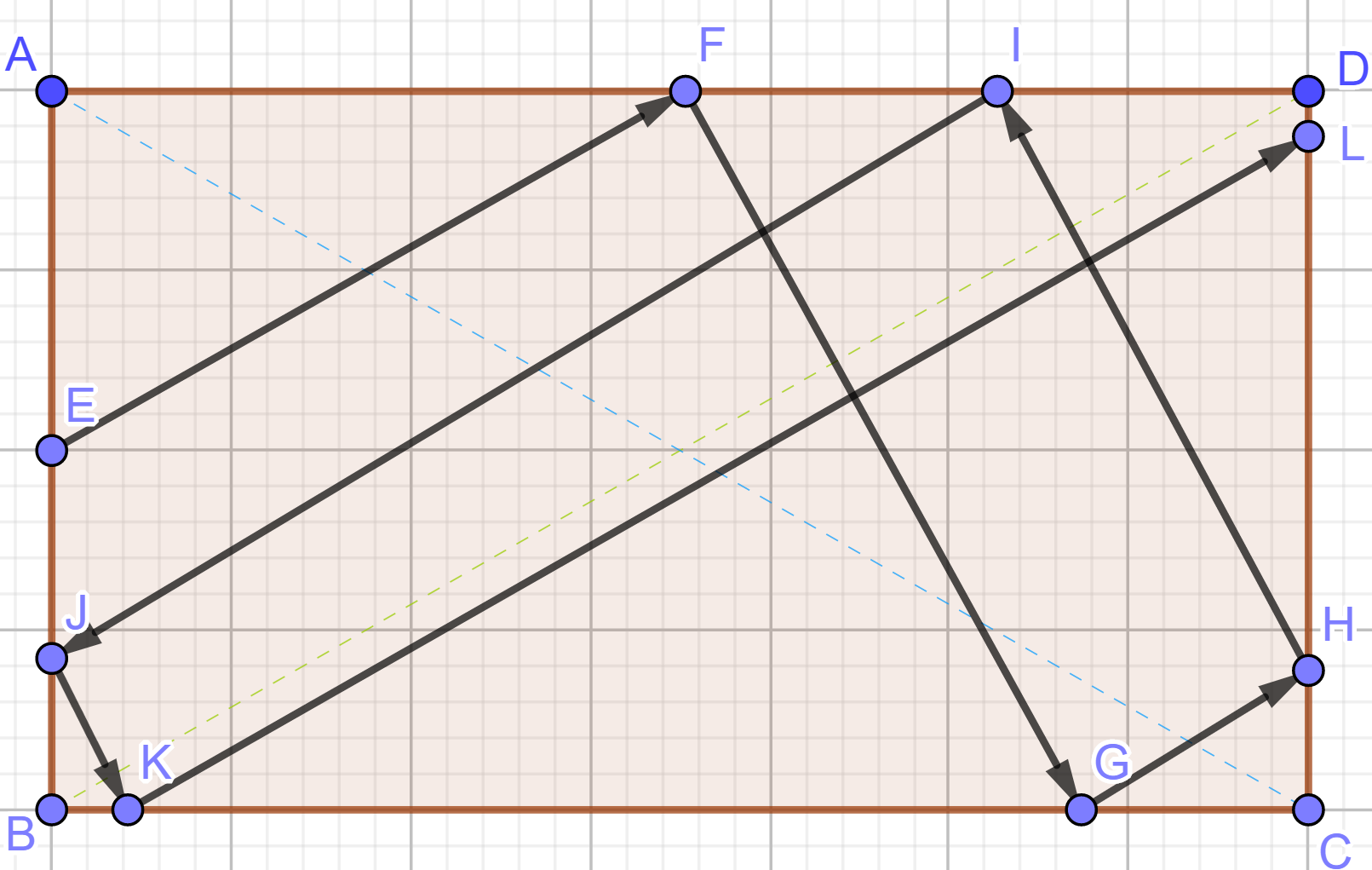## 15. [三角形中线问题]

Posted by haifeng on 2021-01-24 19:49:44 last update 2021-01-24 22:15:42 | Answers (1) | 收藏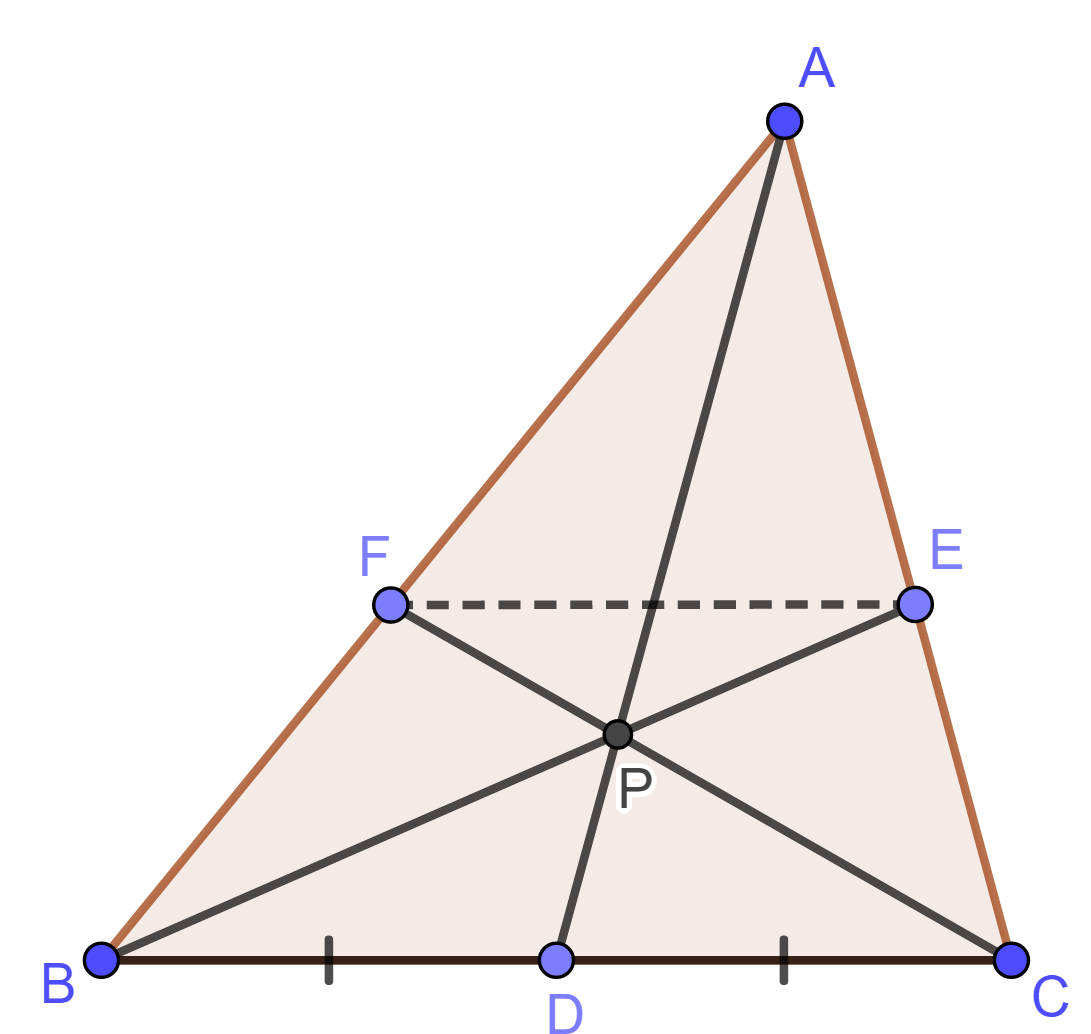【扩展问题1】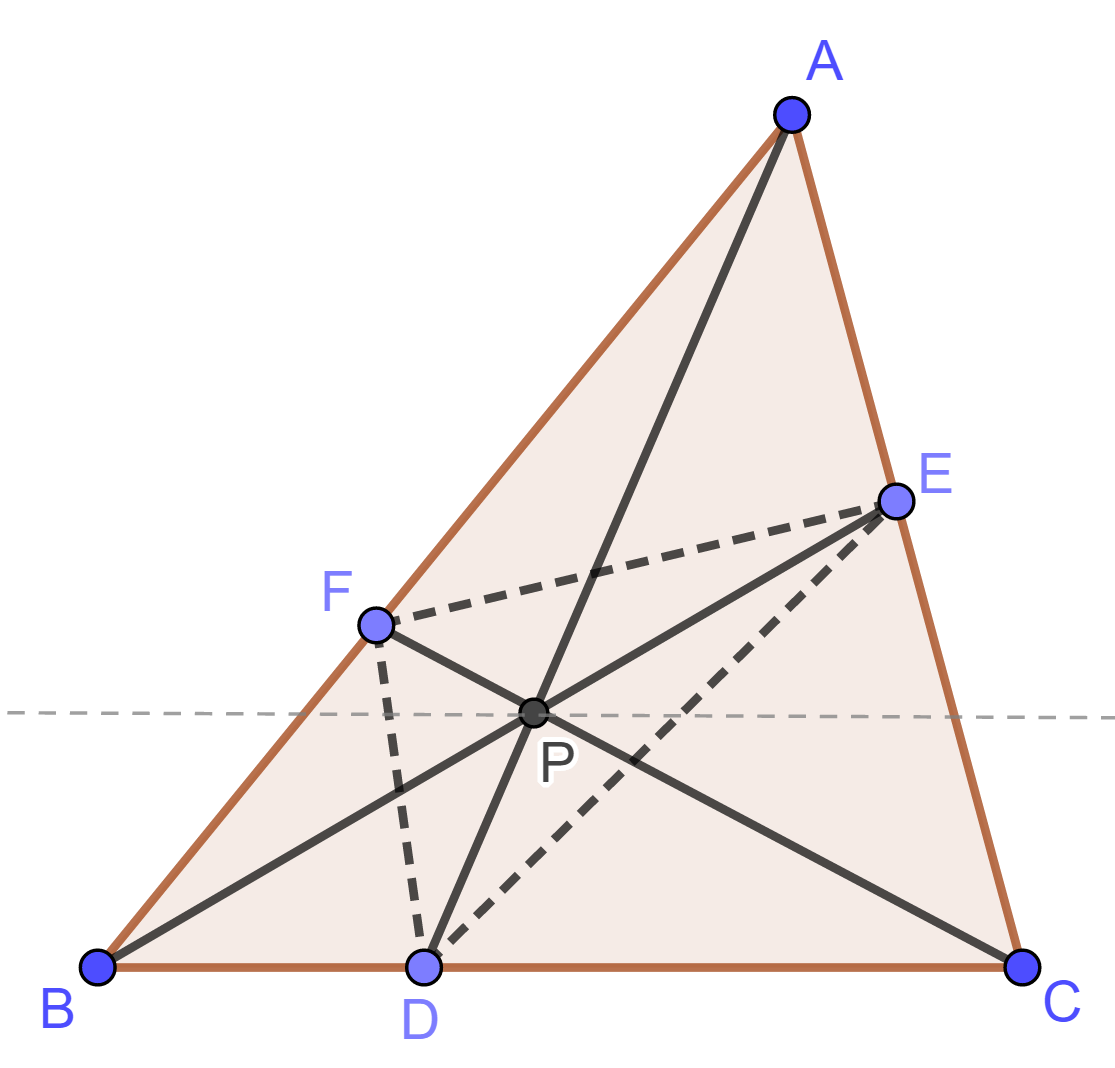【扩展问题2】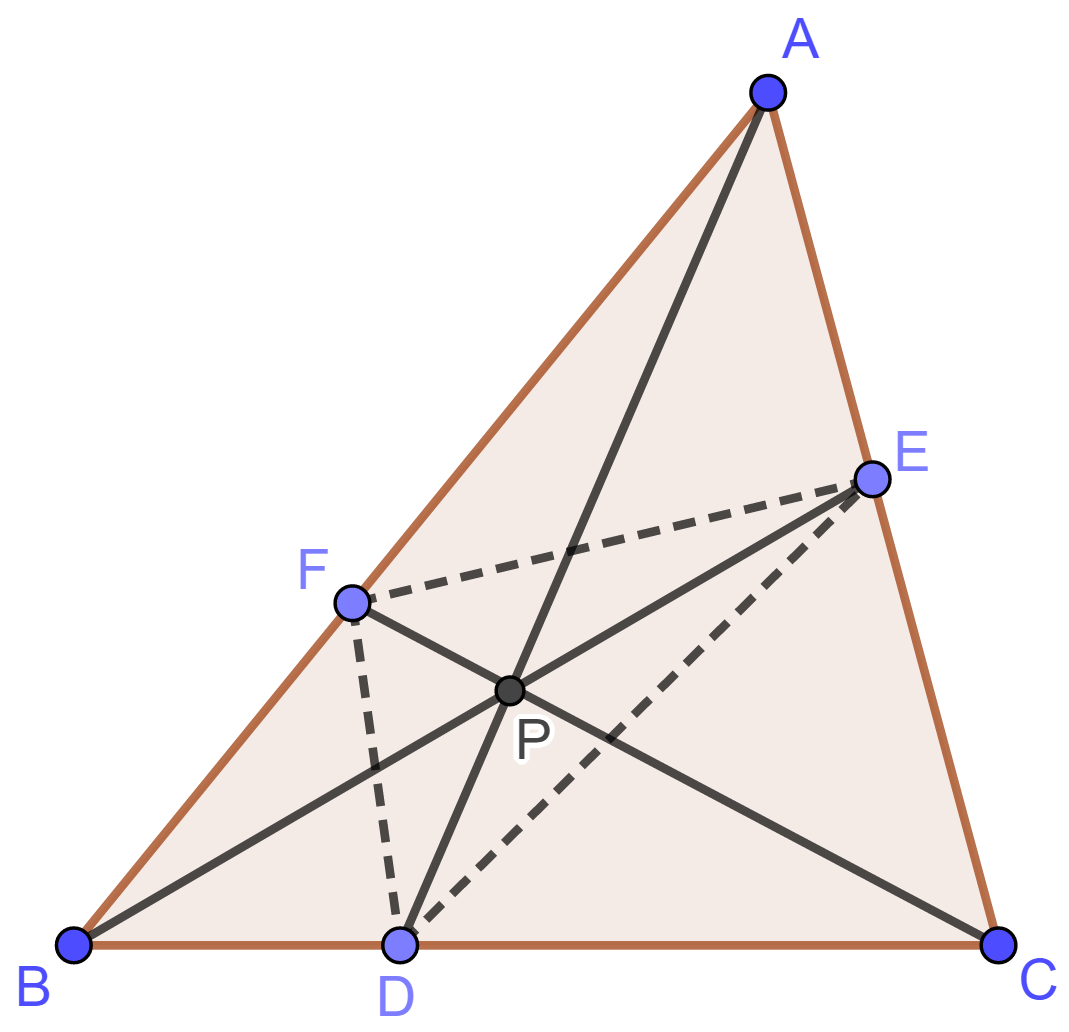## 16. 写出椭圆方程 $\dfrac{x^2}{a^2}+\dfrac{y^2}{b^2}=1$ 的极坐标形式.

Posted by haifeng on 2020-12-04 10:27:17 last update 2020-12-04 10:27:36 | Answers (1) | 收藏

$\rho^2=\frac{a^2 b^2}{a^2\cos^2\theta+b^2\sin^2\theta}$

## 17. 将三角形两边往外绘制两个正方形, 正方形的相应顶点连接. 其中点作三角形第三边的垂线, 证明其平分之.

Posted by haifeng on 2020-05-14 22:23:55 last update 2020-05-14 22:25:30 | Answers (0) | 收藏## 18. 椭圆、双曲线、抛物线的标准方程及它们的焦点和准线

Posted by haifeng on 2019-12-05 21:19:36 last update 2019-12-05 21:19:36 | Answers (0) | 收藏

$\frac{x^2}{a^2}+\frac{y^2}{b^2}=1.$

$\frac{x^2}{a^2}-\frac{y^2}{b^2}=1.$

$y^2=2px,\quad\text{或}\quad x^2=2py$

## 19. 设 $\triangle ABC$ 中 $\angle CAB=60^{\circ}$, $AD$ 是 $\angle CAB$ 的角平分线. 并且 $|AC|=1$, $|AB|=3$. 试求 $|AD|$ 的长.

Posted by haifeng on 2019-01-17 22:47:25 last update 2019-01-17 22:48:43 | Answers (0) | 收藏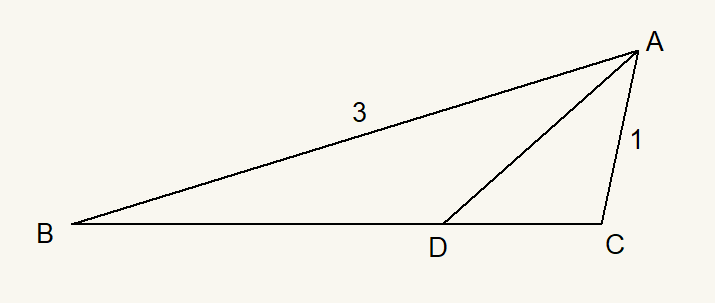[hint] 参考问题2191

## 20. 将直角三角形 $\triangle EAB, \triangle BFC, \triangle CDA$ 如下图放置, 其中 $\angle EAB=\angle BFC=\angle CDA=90^{\circ}$

Posted by haifeng on 2019-01-17 22:03:48 last update 2019-01-17 22:20:20 | Answers (0) | 收藏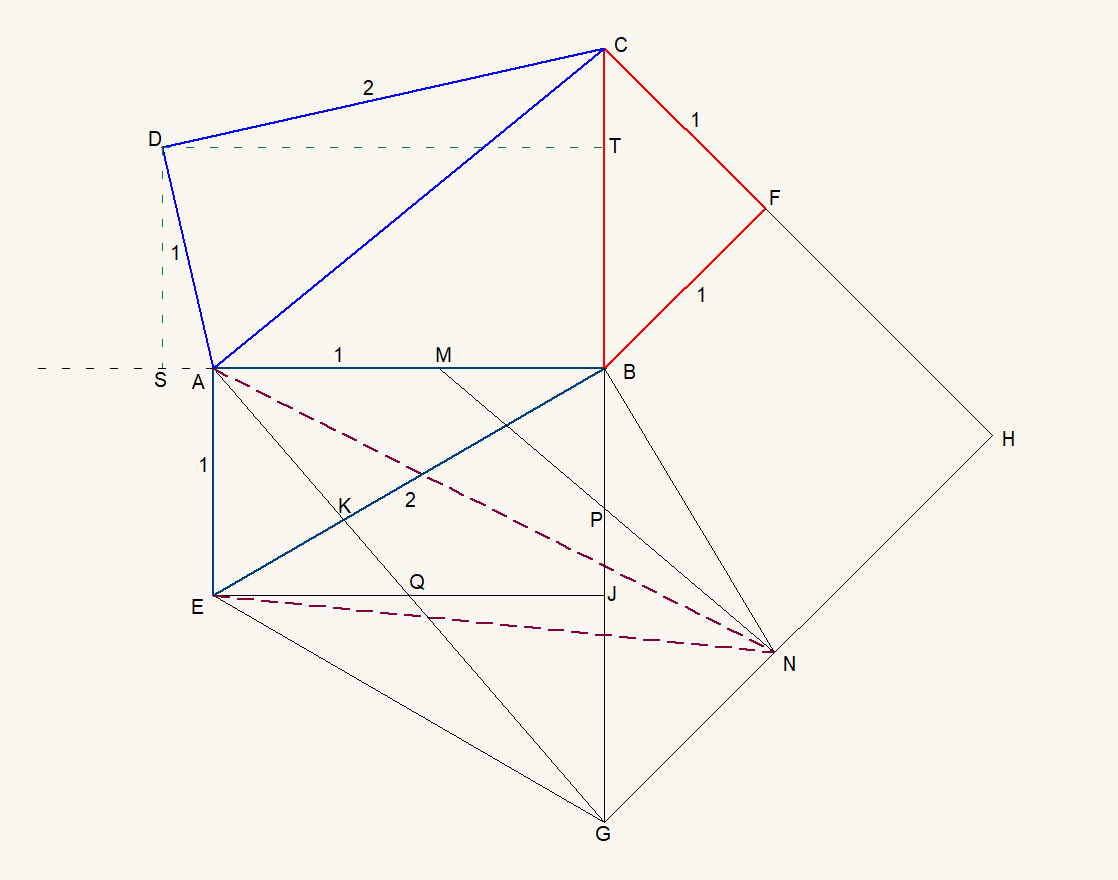• 设 $|AE|=|BF|=|CF|=|DA|=1$,  $|EB|=|CD|=2$.
• 延长 $CB$ 至 $G$, 使得 $|BG|=2$;
• 延长 $CF$ 至 $H$, 使得 $GH\perp CH$.
• 连接 $EG$, $AG$.
• 过点 $B$ 作 $BN\perp EB$, 且 $BN$ 交 $GH$ 于 $N$.
• 在 $AB$ 上取点 $M$, 使得 $|AM|=1$. 连接 $MN$.
• $EJ$ 平行于 $AB$, 且 $EJ$ 交 $AG$ 于点 $Q$.
• 过点 $D$ 作 $DT\perp BC$ 于 $T$, 作 $DS\perp BA$ 延长线 于 $S$.

(1) $\triangle AEG$ 与 $\triangle MBN$ 相似.

(2) $\frac{|AN|}{|EN|}=\frac{\sqrt{5}}{2}$.

(3) $|DT|=2|DS|$.

<   >